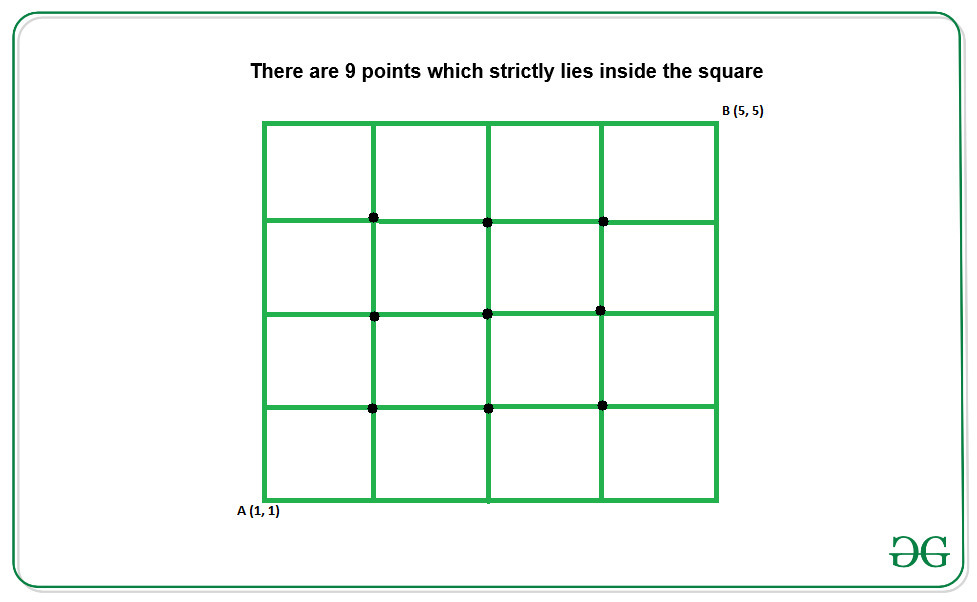# Count of integral coordinates that lies inside a Square

• Last Updated : 24 May, 2022

Given lower left and upper right coordinates (x1, y1) and (x2, y2) of a square, the task is to count the number of integral coordinates that lies strictly inside the square.
Examples:

Input: x1 = 1, y1 = 1, x2 = 5, x3 = 5
Output:
Explanation:
Below is the square for the given coordinates:Input: x1 = 1, y1 = 1, x2 = 4, x3 = 4
Output:

Approach: The difference between the x and y ordinates of the lower and upper right coordinates of the given squares gives the number integral points of x ordinates and y ordinates between opposite sides of square respectively. The total number of points that strictly lies inside the square is given by:

count = (x2 – x1 – 1) * (y2 – y1 – 1)

For Example:In the above figure:
1. The total number of integral points inside base of the square is (x2 – x1 – 1)
2. The total number of integral points inside height of the square is (y2 – y1 – 1)
These (x2 – x1 – 1) integrals points parallel to the base of the square repeats (y2 – y1 – 1) number of times. Therefore the total number of integral points is given by (x2 – x1 – 1)*(y2 – y1 – 1)

Below is the implementation of the above approach:

## C++

 `// C++ program for the above approach``#include ``using` `namespace` `std;` `// Function to calculate the integral``// points inside a square``void` `countIntgralPoints(``int` `x1, ``int` `y1,``                        ``int` `x2, ``int` `y2)``{``    ``cout << (y2 - y1 - 1) * (x2 - x1 - 1);``}` `// Driver Code``int` `main()``{``    ``int` `x1 = 1, y1 = 1;``    ``int` `x2 = 4, y2 = 4;` `    ``countIntgralPoints(x1, y1, x2, y2);``    ``return` `0;``}`

## Java

 `// Java program for the above approach` `class` `GFG {` `// Function to calculate the integral``// points inside a square``static` `void` `countIntgralPoints(``int` `x1, ``int` `y1,``                               ``int` `x2, ``int` `y2)``{``    ``System.out.println((y2 - y1 - ``1``) *``                       ``(x2 - x1 - ``1``));``}` `// Driver Code``public` `static` `void` `main(String args[])``{``    ``int` `x1 = ``1``, y1 = ``1``;``    ``int` `x2 = ``4``, y2 = ``4``;``    ` `    ``countIntgralPoints(x1, y1, x2, y2);``}``}` `// This code is contributed by rutvik_56`

## Python3

 `# Python3 program for the above approach` `# Function to calculate the integral``# points inside a square``def` `countIntgralPoints(x1, y1, x2, y2):``    ``print``((y2 ``-` `y1 ``-` `1``) ``*` `(x2 ``-` `x1 ``-` `1``))` `# Driver Code``if` `__name__ ``=``=` `'__main__'``:` `    ``x1 ``=` `1``    ``y1 ``=` `1``    ``x2 ``=` `4``    ``y2 ``=` `4` `    ``countIntgralPoints(x1, y1, x2, y2)` `# This code is contributed by Samarth`

## C#

 `// C# program for the above approach``using` `System;` `class` `GFG{``    ` `// Function to calculate the integral``// points inside a square``static` `void` `countIntgralPoints(``int` `x1, ``int` `y1,``                               ``int` `x2, ``int` `y2)``{``    ``Console.WriteLine((y2 - y1 - 1) *``                      ``(x2 - x1 - 1));``}` `// Driver code``static` `void` `Main()``{``    ``int` `x1 = 1, y1 = 1;``    ``int` `x2 = 4, y2 = 4;``    ` `    ``countIntgralPoints(x1, y1, x2, y2);``}``}` `// This code is contributed by divyeshrabadiya07   `

## Javascript

 ``

Output:

`4`

Time Complexity: O(1), as we are not using any loops.

Auxiliary Space: O(1), as we are not using any extra space.

My Personal Notes arrow_drop_up